# 1617-257/TUT-R-2

We discussed the following on 9/22/16:

(1) What are the dimensions of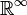$\mathbb{R}^\infty$ and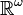$\mathbb{R}^\omega$?

(2) Let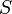$S$ be a subset of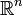$\mathbb{R}^n$. Show that the set of limit points of$S$,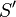$S'$, is closed.

A student gave an for problem (2) which works fine if$S$ is a closed set (it depended on the fact that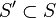$S' \subset S$).

[A student pointed out that I used a definition for limit point which was different from (but also equivalent to) that given in the text. We've replaced any usage of the definition I originally used with the text's definition. We also discussed why the two definitions are equivalent in the Thursday tutorial that took place on 9/29/16.]

Let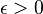$\epsilon > 0$ be given and let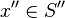$x'' \in S''$ be given.

Then there is some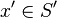$x' \in S'$ such that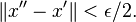$\|x'' - x'\| < \epsilon/2.$

There is also a point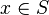$x \in S$ such that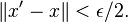$\|x' - x \| < \epsilon/2.$

So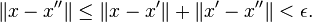$\|x - x''\| \leq \|x - x'\| + \|x' - x''\| < \epsilon.$

That is,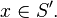$x \in S'.$